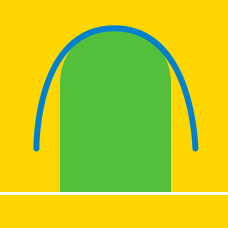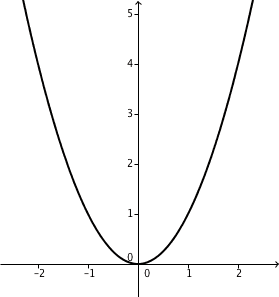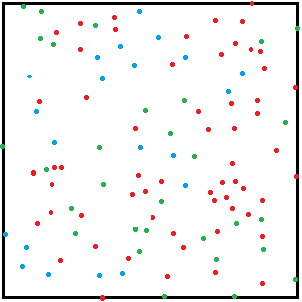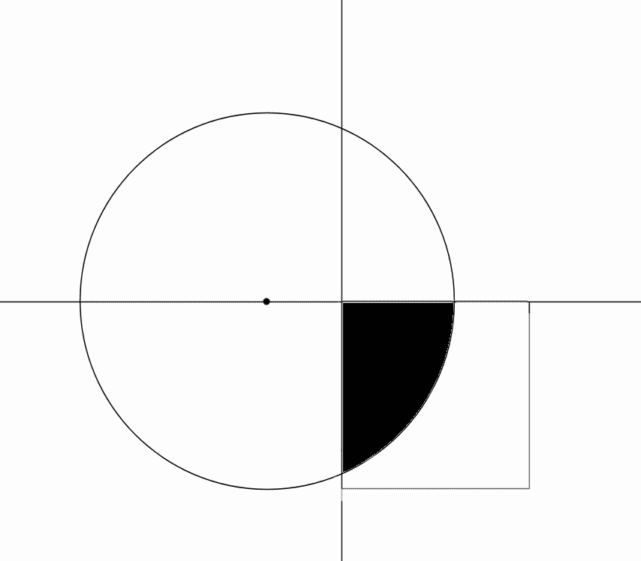Calculus

# Area Between Curves: Level 5 ChallengesThe ends of a stiff bar $\overline{AB}$ of length 4 slide freely inside a parabolic track (specifically, the parabola $y=x^2$). As they do, the midpoint $M$ of that bar traces a curve. Find the area of the region between the parabola and the curve traced by $M$.

Assume that the bar slides infinitely in both directions.If the average distance from a point randomly selected in the unit square to its center equals $\frac{\ln(a+\sqrt{b})+\sqrt{b}}{c}$ where $a,b$ and $c$ are positive integers with $b$ being square-free. Find $a+b+c$.

Let $P$ be a point (other than the origin) lying on the parabola $y = x^{2}$. The normal line to the parabola at $P$ will intersect the parabola at another point $Q$. The minimum possible value for the area bounded by the line $PQ$ and the parabola is $\dfrac{a}{b}$, where $a$ and $b$ are positive coprime integers. Find $a + b$.

Clarification: The normal line is the line perpendicular to the tangent line at a given point on a curve and which passes through the given point.

I have a infinitely long strip of paper that is one unit wide. On the paper is a point $P$ that is $\dfrac{1}{4}$ units away from one of the edges of the strip.

Let $L$ be the locus of all points $O$ such that if I draw the circle with center $O$ passing through $P$, the entire circle can be drawn on the strip of paper. If the area of $L$ can be expressed by $\dfrac{a\sqrt{b}}{c}$ for relatively prime $a,c$ and square-free $b$, then find $a+b+c$.A square $5 \times 5$ is positioned in the $4^\text{th}$ quadrant intersecting part of a circle of radius $5$ and centered at $(-2, 0)$. The square is rotated counter-clockwise about the origin.

Through what angle (in degrees) does the square have to be rotated until $\frac 1 2$ of its area intersects with the circle?

[Answer to nearest 3 decimal places]

×